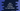# Java program to check if a number is a spy number or not## Java program to check if a number is a spy number or not:

In this post, we will learn how to check if a number is a spy number or not in Java. We will learn the definition of spy number, how to check if a number is spy or not and how to do it in Java.

The program will take one number as input from the user, check if it is a spy number and print one message.

### What is a spy number:

A number is called a spy number if the sum of its digits and the product of its digits are equal. For example,

• 1124 is a spy number because its sum of digits is 1 + 1 + 2 + 4 = 8 and product of its digits is 1 * 1 * 2 * 4 = 8. Both are equal.
• 124 is not a spy number because its sum of digits 1 + 2 + 4 = 7 and product of digits 1 * 2 * 7 = 14 are not equal.

### Algorighm to check if a number is spy number or not:

We will use the below algorithm to check if a given number is a spy number or not:

• Take the number as input from the user.
• Initialize one variables as 0 to hold the sum of the digits and another variable as 1 to find the product of the digits of the number.
• Do the below steps until it become 0:
• Remove the last digit from the number.
• Add it to the sum of digits variable.
• Multiply it with the product of digits variable.
• Once the number is 0, compare the value of sum of all digits with product of all digits.
• If both are equal, print that it is a spy number. Else, print that it is not a spy number.

### Java program:

Let’s write a Java program to check if a number is a spy number or not:

``````import java.util.Scanner;

class Main {
public static void main(String[] args) {
Scanner sc = new Scanner(System.in);
int no, sum = 0, product = 1, lastDigit;

System.out.println("Enter a number: ");
no = sc.nextInt();

while (no > 0) {
lastDigit = no % 10;
sum += lastDigit;
product *= lastDigit;

no /= 10;
}

if (sum == product) {
System.out.println("It is a spy number");
} else {
System.out.println("It is not a spy number");
}

}
}``````

Here,

• sc is a Scanner object to read the user inputs.
• no is an integer variable to hold the user input number. sum is to hold the sum of the digits of the number. It is initialized as 0, product is to hold the product of the digits of the number. It is initialized as 1. lastDigit is to hold the last digit of the number.
• Ask the user to enter a number. Read it and store it in the no variable.
• The while loop runs until the value of no is greater than 0.
• Find the last digit of the number by using modulo operator and store that in the lastDigit variable.
• Add the last digit to the sum variable.
• Multiply the last digit to the product variable.
• Remove the last digit from the number by dividing the number by 10.
• The last if-else condition is checking if the sum is equal to product or not. If both are equal, print that it is a spy number. Else, print that it is not a spy number.

If you run this program, it will print output as like below:

``````Enter a number:
1124
It is a spy number

Enter a number:
12345
It is not a spy number``````

### Java program to check if a number is spy number or not using a different method:

We can also use a different method to check if a number is spy or not. This is basically the same process. The only difference is that it will do the checking in a separate method instead of doing it inside main.

If you put the method in a different class and make the method static, it will work and you can call it from different places of your app.

``````import java.util.Scanner;

class Main {

public static boolean isSpyNumber(int no) {
int sum = 0, product = 1, lastDigit;
while (no > 0) {
lastDigit = no % 10;
sum += lastDigit;
product *= lastDigit;

no /= 10;
}

return sum == product;
}

public static void main(String[] args) {
Scanner sc = new Scanner(System.in);
int no;

System.out.println("Enter a number: ");
no = sc.nextInt();

if (isSpyNumber(no)) {
System.out.println("It is a spy number");
} else {
System.out.println("It is not a spy number");
}

}
}``````

Here,

• We added a new method isSpyNumber that takes one integer value as the parameter and returns one boolean value.
• We are calling this method and passing the user input number in the if statement. If the user input number is a spy number, it will return true and it will run the if block.
• Else, it will run the else block and print that it is not a spy number.

If you run this program, it will print similar result.

``````Enter a number:
1234
It is not a spy number``````